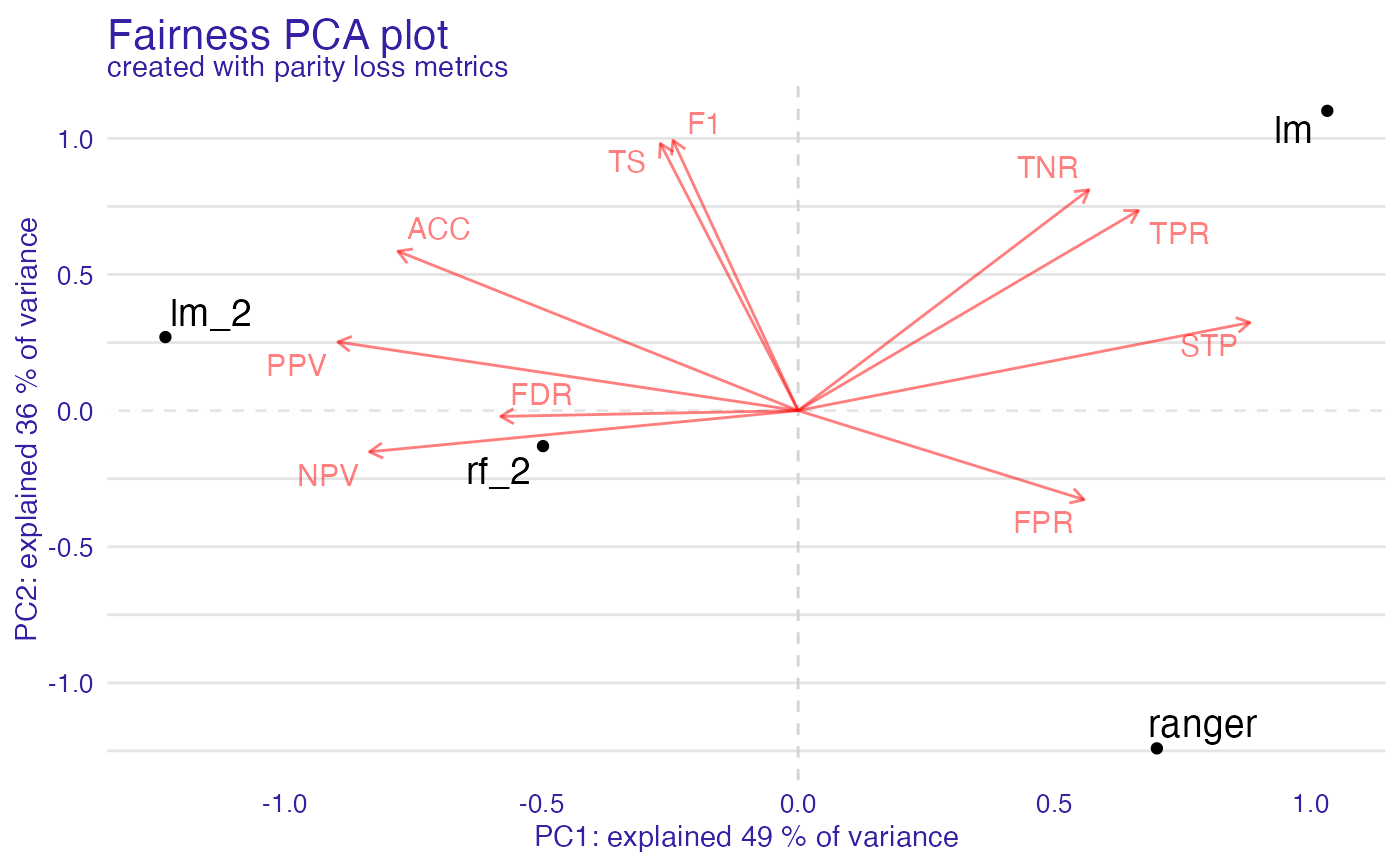Calculate PC for metric_matrix to see similarities between models and metrics. If omit_models_with_NA is set to TRUE models with NA will be omitted as opposed to default behavior, when metrics are omitted.

fairness_pca(x, omit_models_with_NA = FALSE)

Arguments

x object of class fairness object logical, if TRUE omits rows in metric_matrix, else omits columns (default)

Value

fairness_pca object It is list containing following fields:

Examples

data("german")

y_numeric <- as.numeric(german$Risk) - 1 lm_model <- glm(Risk ~ ., data = german, family = binomial(link = "logit") ) rf_model <- ranger::ranger(Risk ~ ., data = german, probability = TRUE, num.trees = 200, num.threads = 1 ) explainer_lm <- DALEX::explain(lm_model, data = german[, -1], y = y_numeric) #> Preparation of a new explainer is initiated #> -> model label : lm ( default ) #> -> data : 1000 rows 9 cols #> -> target variable : 1000 values #> -> predict function : yhat.glm will be used ( default ) #> -> predicted values : No value for predict function target column. ( default ) #> -> model_info : package stats , ver. 4.1.1 , task classification ( default ) #> -> predicted values : numerical, min = 0.1369187 , mean = 0.7 , max = 0.9832426 #> -> residual function : difference between y and yhat ( default ) #> -> residuals : numerical, min = -0.9572803 , mean = 1.940006e-17 , max = 0.8283475 #> A new explainer has been created! explainer_rf <- DALEX::explain(rf_model, data = german[, -1], y = y_numeric) #> Preparation of a new explainer is initiated #> -> model label : ranger ( default ) #> -> data : 1000 rows 9 cols #> -> target variable : 1000 values #> -> predict function : yhat.ranger will be used ( default ) #> -> predicted values : No value for predict function target column. ( default ) #> -> model_info : package ranger , ver. 0.13.1 , task classification ( default ) #> -> predicted values : numerical, min = 0.05815476 , mean = 0.6969663 , max = 0.9975069 #> -> residual function : difference between y and yhat ( default ) #> -> residuals : numerical, min = -0.7217679 , mean = 0.003033675 , max = 0.6242611 #> A new explainer has been created! fobject <- fairness_check(explainer_lm, explainer_rf, protected = german$Sex,
privileged = "male"
)
#> Creating fairness classification object
#> -> Privileged subgroup		: character ( Ok  )
#> -> Protected variable		: factor ( Ok  )
#> -> Cutoff values for explainers	: 0.5 ( for all subgroups )
#> -> Fairness objects		: 0 objects
#> -> Checking explainers		: 2 in total (  compatible  )
#> -> Metric calculation		: 10/13 metrics calculated for all models ( 3 NA created )
#>  Fairness object created succesfully

# same explainers with different cutoffs for female
fobject <- fairness_check(explainer_lm, explainer_rf, fobject,
protected = german\$Sex,
privileged = "male",
cutoff = list(female = 0.4),
label = c("lm_2", "rf_2")
)
#> Creating fairness classification object
#> -> Privileged subgroup		: character ( Ok  )
#> -> Protected variable		: factor ( Ok  )
#> -> Cutoff values for explainers	: female: 0.4, male: 0.5
#> -> Fairness objects		: 1 object (  compatible  )
#> -> Checking explainers		: 4 in total (  compatible  )
#> -> Metric calculation		: 10/13 metrics calculated for all models ( 3 NA created )
#>  Fairness object created succesfully

fpca <- fairness_pca(fobject)
#> Warning: Found metric with NA: FNR, FOR, NEW_METRIC, omiting it

plot(fpca)